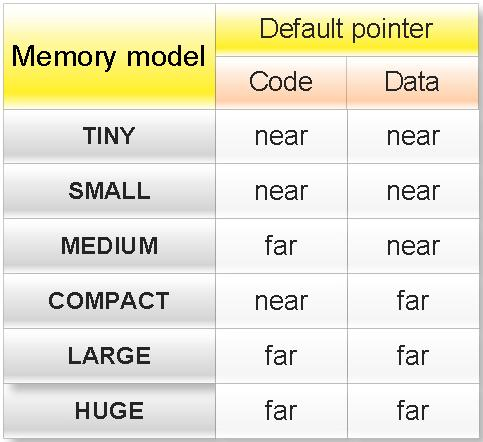## Pages

### Memory Model in C Programming

Memory model:

In c there are six type of memory model.
If you want to see all memory model in Turbo C++ IDE then open Turbo C++ IDE and the go:
Options menu -> Compiler -> Code generation
These memory models are:
(a) TINY
(b) SMALL
(c) MEDIUM
(d) COMPACT
(e) LARGE
(f) HUGE

If you want to change the memory model then go to:
Options menu -> Compiler -> Code generation
And select any memory model and click OK button.Properties of memory mode in C:

(1) Memory model decides the default type of pointer in C.

Note:
Code: A pointer to function is called code.
Data: A pointer to variable is called data.

Examples:

(1)What will be output of following c program?

void main(){
int *ptr;
printf("%d",sizeof ptr);
}

Output: Depends upon memory model.

Explanation: If memory model is TINY, SMALL or MEDIUM then default pointer will near and output will be 2 other wise output will be 4.

(2)What will be output of following c program?

void main(){
char (*fun)();
printf("%d",sizeof fun);
}

Output: Depends upon memory model.

Explanation: fun is pointer to function. If memory model is TINY, SMALL or COMPACT then default pointer will near and output will be 2 other wise output will be 4.

(3)What will be output of following c program?

void main(){
int near *p,*q;
printf("%d , %d",sizeof(p),sizeof(q));
}

Output: 2, Depend upon memory model.

Explanation: p is near pointer while type of pointer q will depend what is default type of pointer.

(4)What will be output of following c program?

void main(){
char huge **p;
printf("%d , %d",sizeof(p),sizeof(*p));
}

Output: 4, Depend upon memory model.

Explanation: p is huge pointer while type of pointer *p will depend what is default type of pointer.

(5)Write a c program to find the memory model of you computer?

void main(){
#if defined __TINY__
printf("Memory model is: TINY");
#elif defined __SMALL__
printf("Memory model is:SMALL ");
#elif defined __MEDIUM__
printf("Memory model is:MEDIUM ");
#elif defined __COMPACT__
printf("Memory model is:COMPACT ");
#elif defined __LARGE__
printf("Memory model is:LARGE ");
#elif defined __HUGE__
printf("Memory model is:HUGE ");
#endif
}

(2) Memory models decide the default size of segment.